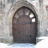Choose Language Hide Translation Bar
Highlighted

## Standard deviation issues under one way ANOVA

Hello all,

I am noticing that when I run a one way ANOVA on my data set, the summary report shows differnet means for my factors but not different values for my standard deviation.

My experiment is looking at the relationship between soil respiration levels and vegetation types. There are 4 vegetation types with 4 replications of each plot. Then I took 3 subsamples from each plot that I will average for 7 visits. SO in total thats 288 data points as of now.

Can anybody help me out?

1 ACCEPTED SOLUTION

Accepted Solutions
Highlightedsusan_walsh1
Staff (Retired)

## Re: Standard deviation issues under one way ANOVA

Rachel,

In the Oneway Analysis platform, if you are looking at the Means for Oneway Anova table, the standard errors reported there are based on the pooled standard deviation used by the analysis of variance. One of the assumptions of this analysis is that the variances are equal.

If you want to see the sample standard deviations calculated based on the data, choose Means and Std Dev from the red triangle menu.

4 REPLIES 4
Highlightedsusan_walsh1
Staff (Retired)

## Re: Standard deviation issues under one way ANOVA

Rachel,

In the Oneway Analysis platform, if you are looking at the Means for Oneway Anova table, the standard errors reported there are based on the pooled standard deviation used by the analysis of variance. One of the assumptions of this analysis is that the variances are equal.

If you want to see the sample standard deviations calculated based on the data, choose Means and Std Dev from the red triangle menu.

Highlighted

## Re: Standard deviation issues under one way ANOVA

Hello Susan,

I am running into a similar situation as Rachel. I would like to see sample standard deviations calculated based on data however I don't see the options you mentioned in the from the red triangle menu. I am running a two-way anova rather than a one-way would that make a difference as to which options I see?

Thanks very much,

Jessica

Highlighted

## Re: Standard deviation issues under one way ANOVA

The previous replies were for a one-way ANOVA performed with the Oneway platform. I assume that you started with Analyze > Fit Model and are now accessing the Fit Least Squares report. This report only provides the pooled standard deviations.

I suggest that you try either Tables > Summary or Analyze > Tabulate to obtain the un-pooled sample standard deviation estimates.

Learn it once, use it forever!
Highlighted

## Re: Standard deviation issues under one way ANOVA

Adding to Sue's guidance, you can use the Unequal Variance command from the platform red triangle menu to test if the group standard deviations are not equal. If you decide that they are different, the Walsh ANOVA is provide, which does not assume pooling.

Learn it once, use it forever!
Article Labels

There are no labels assigned to this post.